Midterm 3 Preparation and Sample Questions

# Midterm 3: Version B

For problems 1–4, perform the indicated operations and simplify.

1.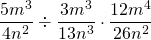2.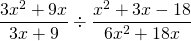3.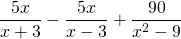4.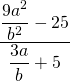Reduce the expressions in questions 5–7.

1.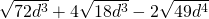2.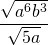3.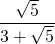Solve for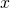.

1.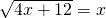For problems 9–12, find the solution set by any convenient method.

1.1.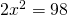2.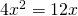2.1.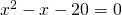2.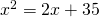3.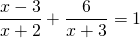4.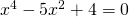5. The length of a rectangle is 3 m longer than its width. If it has a perimeter that is 46 m long, then find the length and width of this rectangle.
6. Find three consecutive even integers such that the product of the first two is 16 more than the third.
7. A boat cruises upriver for 4 hours and returns to its starting point in 2 hours. If the speed of the river is 5 km/h, find the speed of this boat in still water.

<a class=”internal” href=”/intermediatealgebraberg/back-matter/midterm-3-version-b/”>Midterm 3: Version B Answer Key